Home » BASIC PROGRAMMING 2

# BASIC PROGRAMMING 2BASIC MATHEMATICAL FUNCTIONS

The BASIC language will allow the following functions to be used within arithmetic expressions.

1) BASIC ABS Function: The ABS function returns the absolute value of an expression.

Format

ABS(X)

Where

X is a number, numeric variable or numeric expression

For example, ABS (-4.2) = 4.2

2) BASIC EXP Function: The EXP function calculates the value of e raised to the X power, where e is equal to 2.71828. That is, EXP(X) is equivalent to 2.71828 X.

Format

EXP (X)

Where

X is a number, numeric variable, expression, or another function. For example, EXP (1) = 2.71828

3) BASIC INT Function: The INT function returns the value of the largest integer not greater than the argument.

Format

INT(X)

Where

X is a number, numeric variable, expression, or another function. For example, INT (2.1) = 2

This function can be used to round numbers to the nearest integer by specifying INT(X+0.5).

For example, the function INT (34.67) has the value 34; the functions INT(34.67+.5) and INT(34.37+.5) have these values of 35 and 34, respectively; and these functions INT(-23) and INT(- 14.39) have these values of – 23 and – 15, respectively.

4) BASIC RND Function: The RND function produces random numbers between (but not including O and 1).

Format

RND(X)

where

X is a dummy variable in this function. RND returns the next pseudo – random number in a uniformly distributed set of numbers in a range. 0 <= RND (X) < 1

5) BASIC SGN Function: The SGN function creates a value based on the sign of the argument. Format

SGN(X)

Where

X is a number, numeric variable, numeric expression, or another function.

The value of the SGN function will be 1 if the argument is any positive number, 0 if the argument is zero and – 1 if the argument is negative.

For example, SGN (+23.1) = 1

6) BASIC SQR Function: The SQR function computes the positive square root of an expression. X must not be negative.

Format

SQR (X)

Where

X is a number, numeric variable, numeric expression, or another function.

7) BASIC ATN Function: The ATN function calculates the angle (in radians) whose tangent is given as the argument of the function.

Format

ATN(X)

Where

X is a number, numeric variable, expression, or another function, representing the tangent of an angle.

For example, ATN(1) = 0.7853982, since the tangent of π/4 is 1.

8) BASIC COS Function: The COS function is used to calculate the cosine of an angle specified in radians.

Format

COS(X)

Where

X is a number, numeric variable, expression, or another function, representing the size of an angle in radians.

For example: COS (0.78539818) = 0.7071

Note: /4 0.78539818

9) BASIC LOG Function: The LOG function calculates the natural logarithm of X (to the base e). Format

LOG(X)

Where

X is a number, numeric variable, expression, or another function.

10) BASIC SIN Function: The SIN function is used to calculate the sine of an angle specified in radians.

Format

SIN(X)

Where

X is a number, numeric variable, expression or another function, representing the size of an angle in radians.

For example: SIN (1.0472) =0.866

Note: /3 1.0472

The magnitude in radians of one complete revolution (360 degrees) is the length of the entire circumference divided by the radius, or 2πr /r, or 2π. Thus 2π radians is equal to 360 degrees, meaning that one radian is equal to 180/π degrees.

One radian is equal to 180/π degrees. Thus, to convert from radians to degrees, multiply by 180/π.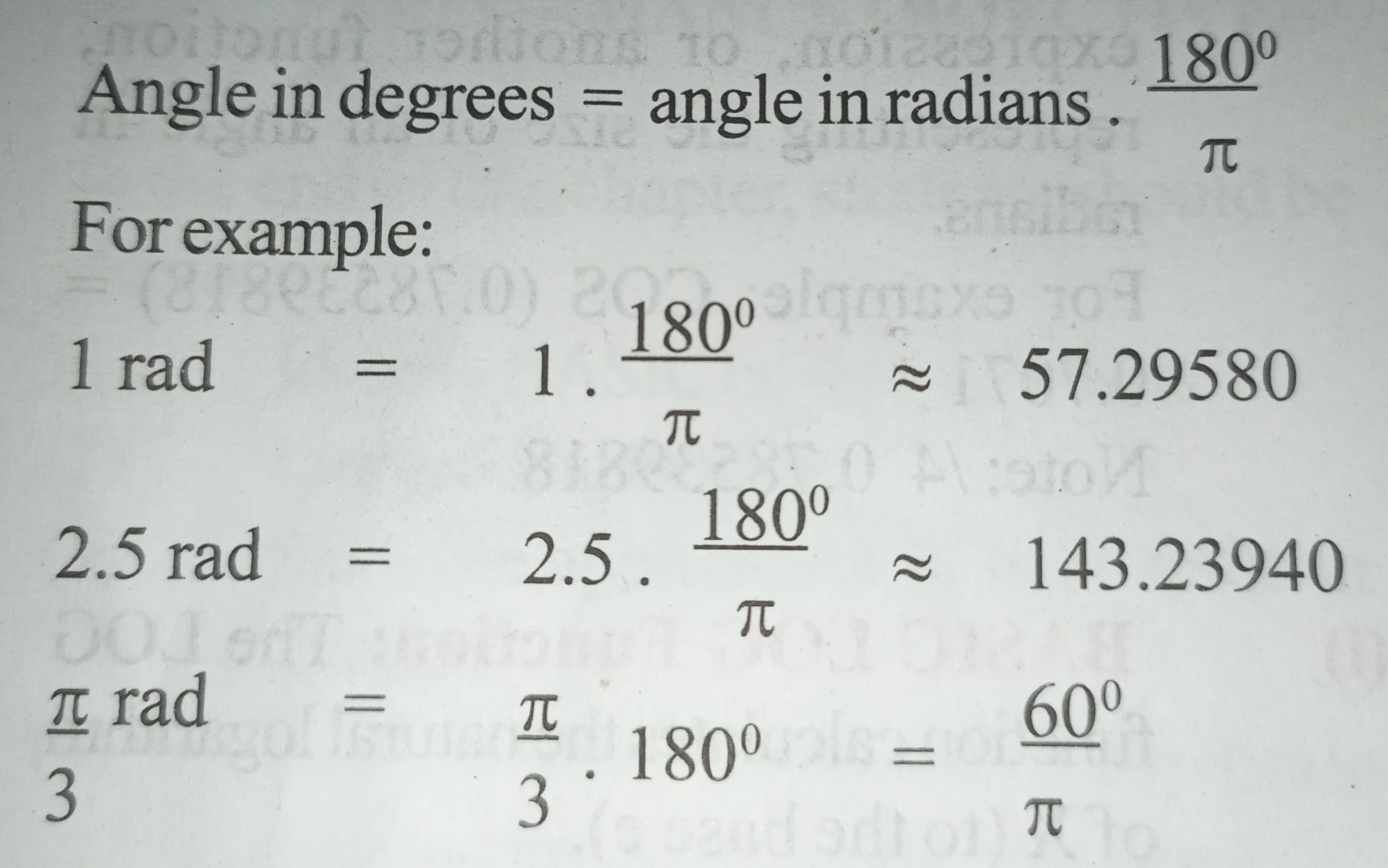Conversely, to convert from degrees to radians, multiply by π/180.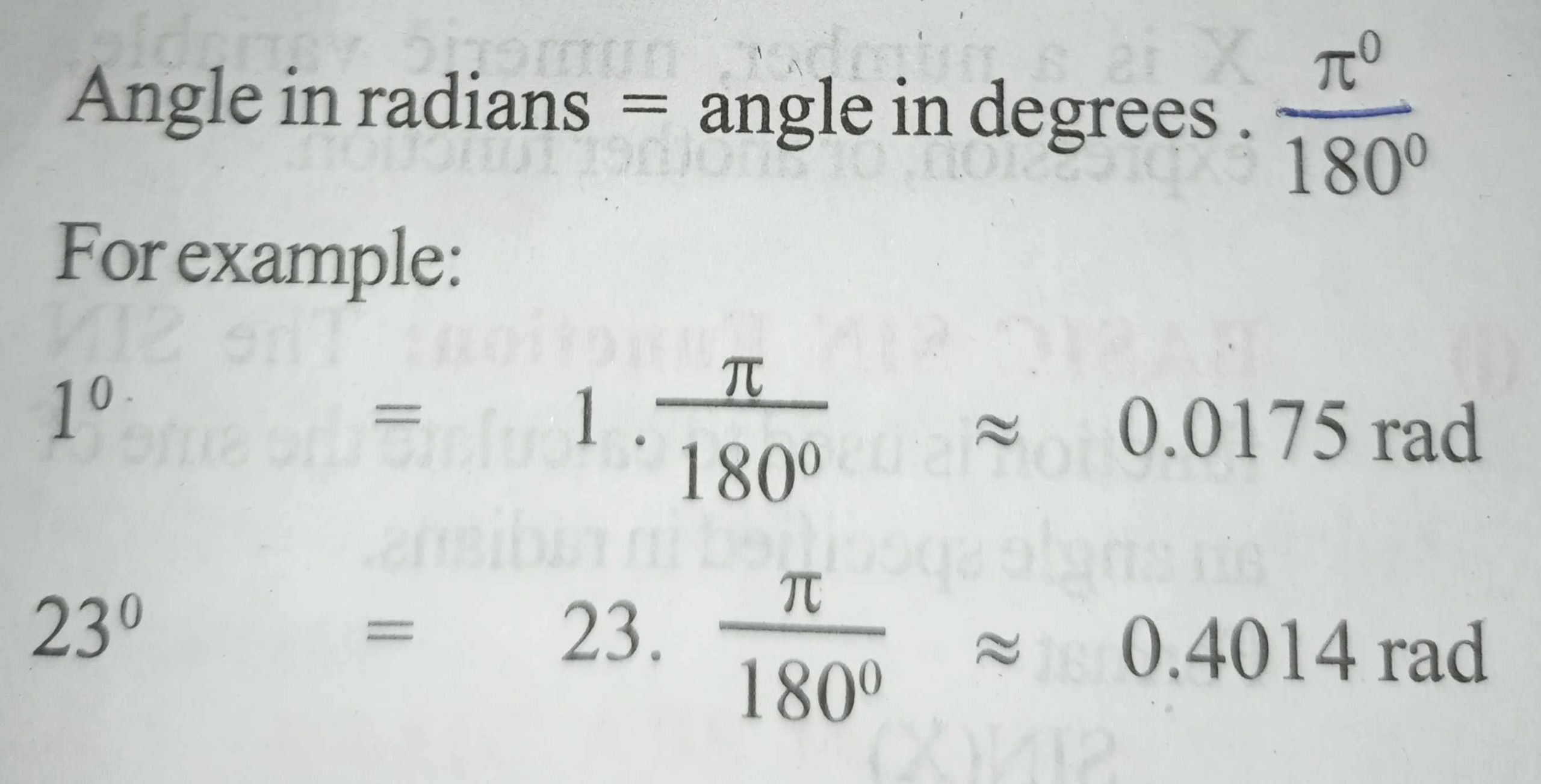Radians can be converted to turns by dividing the number of radians by 2π

We know that the length of circumference of a circle is given by 2πr , where r is the radius of the circle.

So, we can very well say that the following equivalent relation is true:

360°⇔ 2πr[Since a 360° sweep is need to draw a full circle].

By definition of radian, we can formulate that a full circle represents:

Combining both the above relations we can say: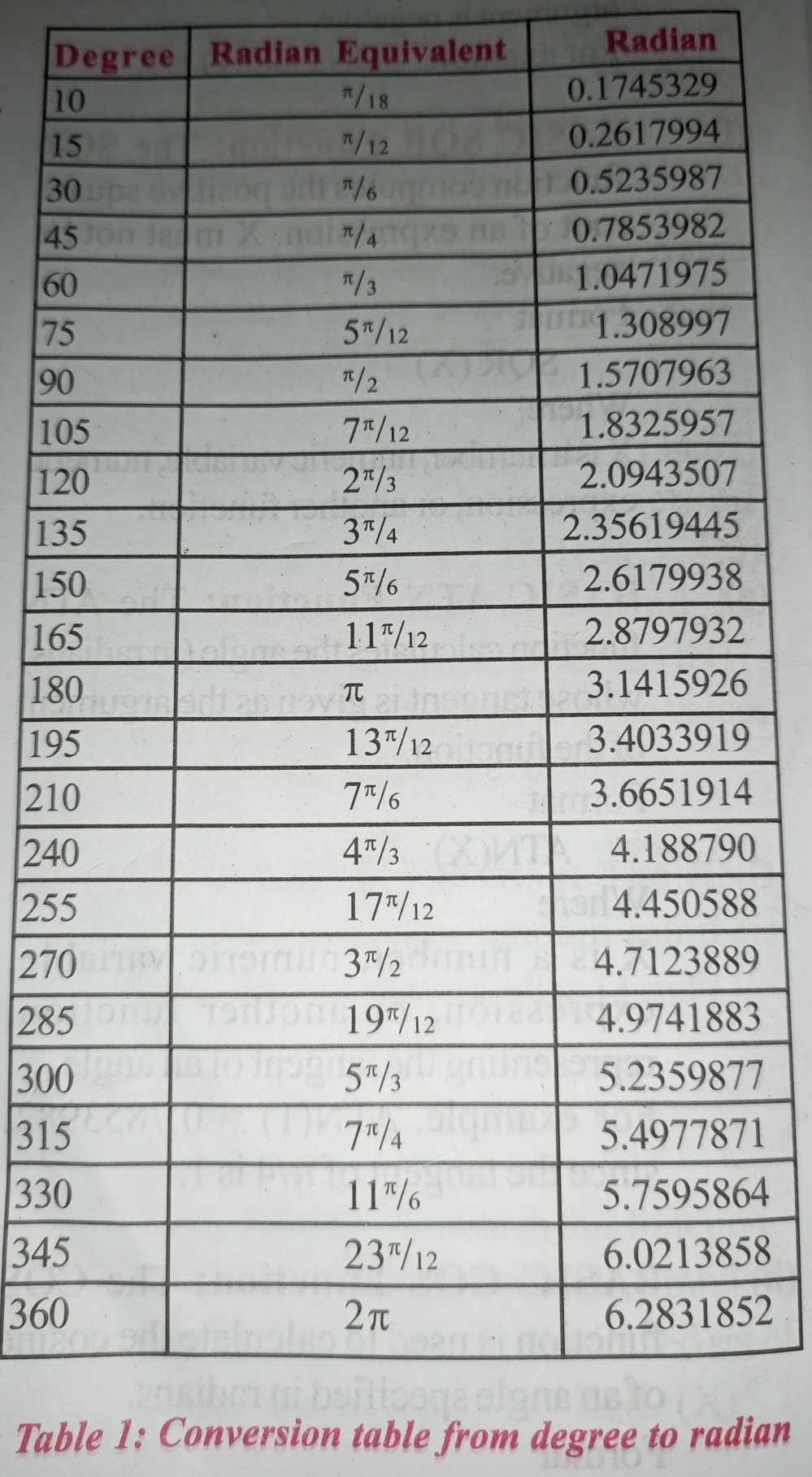TRIGONOMETRIC FUNCTION GRAPH ON BASIC PROGRAMMING LANGUAGE

In plotting the trigonometric function graph on BASIC, the program should display a horizontal axis to represent the angle X, and the vertical axis to represent the function of the graph. The graph should be store in a two dimensional table having 21 rows and 51 columns. Each cell of the table been used to store a single character. Before the coordinates of the graph can be stored in the table each cell must be initialized with a space character, then the vertical axis with scale and horizontal axis can be stored.

Using the trigonometric functions, the coordinates of the graph can be used as subscripts to the table and an ”*” character can be stored to represent the plot of the function. When the plotting of characters is completed, the contents of the table can be printed row by row, and finally the horizontal scale printed at the bottom of the graph. Since the functions Sin (X) and Cos (X) will only produce values in the range 1 to 1inclusive, it is necessary to convert the values 1 to 21 inclusive on the table. Thus, the ordinates (+1) is transformed to row 1, and the ordinate (-1) is transformed to row 21. Intermediate ordinates must also be transformed to row value. To achieving this transformation, it is necessary to define two functions.

Worked Examples

1) Write a BASIC program to find the square root of numbers from 10 to 50.

Solution

10 REM Program to calculate the square root of numbers

20 PRINT “NUMBER”, “SQUARE ROOT”

30 FOR N = 10 TO 50 STEP 1

40 LET Q = SQR (N)

50 PRINT N, Q

60 NEXT N

70 STOP

2) Write a program to print the values of LOG (X) and EXP (X) for X varying between 1 and 10 in steps of 0.5.

Solution

10 REM VALUES OF LOG(X) AND EXP (X)

20 PRINT “X”, “LOG(X)”, “EXP(X)”

30 FOR X = 1 TO 10 STEP 0.5

40 REM CALCULATE THE VALUE OF LOG(X) AND EXP(X)

50 LET A = LOG(X)

60 LET B= EXP(X)

70 PRINT X, A, B

80 NEXT X

90 STOP

3) Write a program to evaluate Sin²x + Cos²x – 1 and Tan²x – Sec²x +1 for values of X between 0 and 1 in steps of 0.1 radians.

Solution

10 REM EVALUATING THE VALUES OF TRIGONOMETRIC FUNCTIONS

20 PRINT “X”, “Y”, “Z”

30 FOR X = 0 TO 1 STEP 0.1

40 LET Y = SIN(X)^2 + COS(X)^2-1

50 LET Z = TAN(X)^2 – (1/(COS(X)^2)) + 1

60 PRINT X, Y, Z

70 NEXT X

80 STOP

4)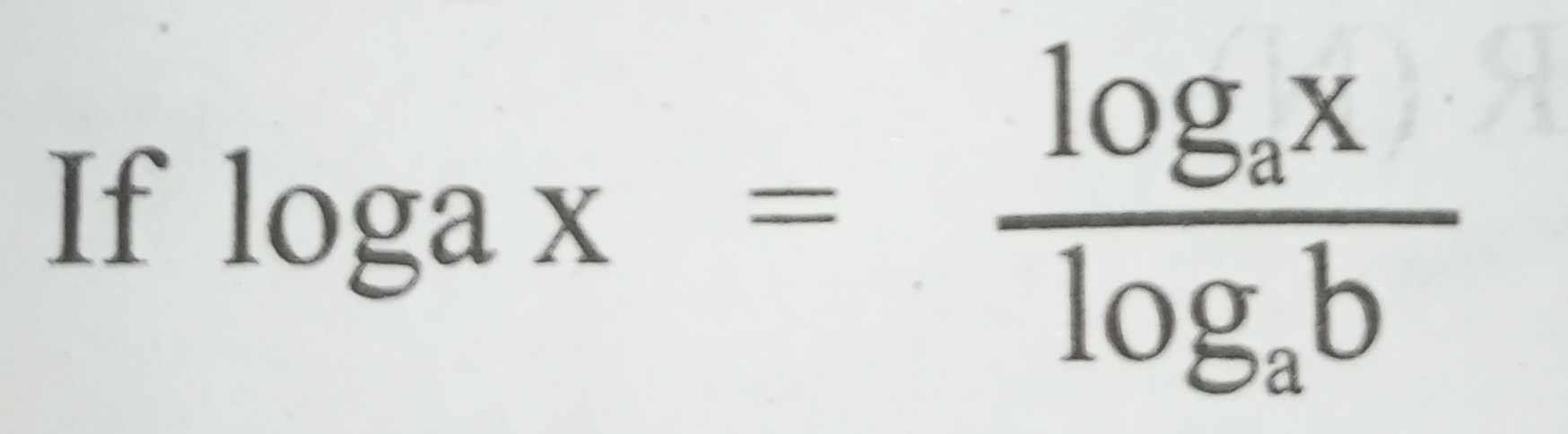then derive a user defined function to calculate the logarithm of a number of any valid given base. Use this function in writing a computer program to find the logarithms of the numbers from 2 to 10 in steps of 0.5 to the base 2 to 10 in steps of 2. Your output should be presented in the form of a table having the numbers down the page and the bases across the page.

Solution

10 REM PROGRAM TO EVALUATE LOGARITHM FUNCTION

20 DEF FNL (A) = LOG(A) / LOG(B)

30 PRINT “NUMBERS”, “BASES”

40 PRINT TAB(10); “2” ; TAB(25); “4” ; TAB(40); “6”;

50 PRINT TAB(55); “8”; TAB(70); “10”

60 FOR A = 2 TO 10 STEP 0.5

70 PRINT A

80 FOR B = 2 TO 10 STEP 2

90 PRINT TAB(10 + 15*(B-2)/2); FNL(A);

100 NEXT B

110 PRINT

120 NEXT A

130 STOP

5) Write a program to calculate the roots of a quadratic equation ax² + bx + c = 0 for the formula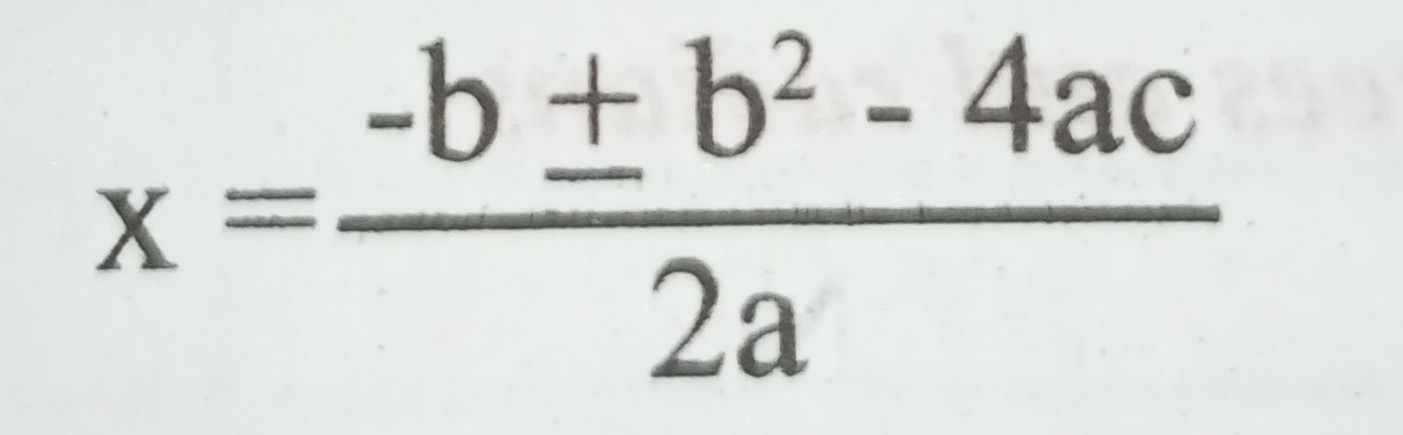The values of a, b, and c being input at the beginning of the program. Consider the implications within your program of b² < 4ac.

Solution

10 REM CALCULATING THE ROOTS OF QUADRATIC EQUATION

20 INPUT “COEFFICIENTS (A, B,C”,A,B,C

30 IF A = 0 THEN STOP

40 LET D = B*B-4*A*C

50 IF D>0 THEN PRINT “REAL DISTINT ROOTS”,

60 LET X1 = (-B+SQR(D))/(2*A)

70 LET X2 = (-B-SQR(D))/(2*A)

80 PRINT “ROOTS ARE”; “X1”; “X2”

90 GOTO 20

100 IF D<0 THEN PRINT “IMAGINARY ROOTS”;

110 STOP

6) Write a program to simulate the rolling of two dice and determine the number of doubles that appear for the digits 1 to 6 inclusive, when the dice are rolled.

(a) 10 times.

(b) 100 times.

(c) 1000 times.

Solution

10 DIM D(6)

20 FOR S=1 TO 3 )

40 DATA 10,100,1000

50 FOR J=1 TO 6

60 D(J) = 0

70 NEXT J

80 FOR R = 1 TO M

90 LET X = INT(6*RND(1)+1)

100 LET Y = INT(6*RND(1)+1)

110 IF X = Y THEN D(X) = D(X) + 1

120 NEXT R

130 PRINT “FOR”;M; “ROLLS OF DICE, DOUBLES ARE:”

140 PRINT

150 PRINT “NUMBER”, “FREQUENCY”

160 FOR J = 1 TO 6

170 PRINT J, D(J)

180 NEXT J

190 NEXT S

200 STOP

7) The line Y = 2X+3 divides the X, Y planes into the two regions. Write a program to input order pairs of numbers that represent coordinates in the X, Y plane. Compute whether the point indicated by the coordinate’s lies above, below or on the line. Print the coordinates indicating the position of the point relative to the line.

Solution

10 INPUT X, Y

20 REM CALCULATE ORDINATE OF Y = 2X + 3

30 LEY Y1=2*X+3

40 REM FIND THE POSITION OF THE POINT RELATIVE TO LINE

50 LET D = Y-Y1

60 PRINT “COORDINATES”;X; “” ; Y;

70 IF D<0 THEN PRINT “BELOW LINE”

80 IF D=O THEN PRINT “ON LINE”

90 IF D>0 THEN PRINT “ABOVE LINE”

100 STOP

8) Write a program to print the values of SINE (X), COSINE (X) and TAN (X) for X varying between 0° and 360° in steps of 15°.

Solution

10 REM VALUES OF SIN(X), COS(X) AND TAN(X)

20 PRINT “X”, “SIN(X)”, “COS(X)”, “TAN(X)

30 FOR X = 0 TO 360 STEP 15

40 LET X1= X*3.14159/180

50 LET A=SIN(X1)

60 LET B = COS(X1)

70 LET C = TAN(X1)

80 PRINT X, A, B,C

90 NEXT X

100 STOP

9) Write a program to evaluate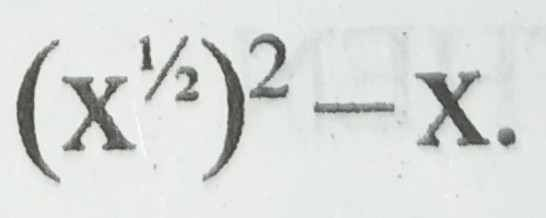0≤X≤1 step 0.05

Solution

10 FOR X = 0 TO 1 STEP 0.05

20 LET Y = (SQR(X))^2-X

30 PRINT X, Y

40 NEXT X

50 STOP

10) Write a program to evaluate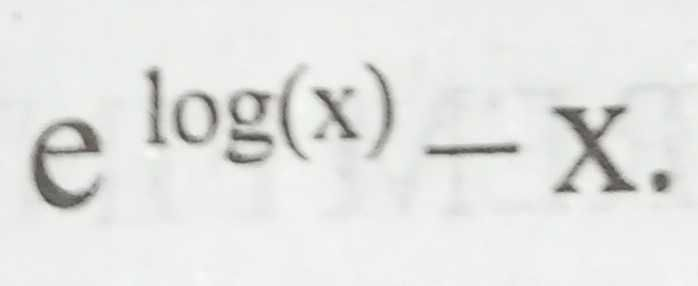1 ≤ x ≤ 10 step 1

Solution

10 FOR X = 1 TO 10 STEP 1

20 LET Y = EXP(LOG(X))-X

30 PRINT X, Y

40 NEXT X

50 STOP

11) Write a program to evaluate tan (arc tan(x)) – x.

-100 ≤ x ≤ 100 100 step 10

Solution

10 FOR X = – 100 TO 100 STEP 10

20 LET Y = TAN (ATN(X))-X

30 PRINT X, Y

40 NEXT X

50 STOP